Courses

# Thermal Noise Electronics and Communication Engineering (ECE) Notes | EduRev

## Communication Theory

Created by: Machine Experts

## Electronics and Communication Engineering (ECE) : Thermal Noise Electronics and Communication Engineering (ECE) Notes | EduRev

The document Thermal Noise Electronics and Communication Engineering (ECE) Notes | EduRev is a part of the Electronics and Communication Engineering (ECE) Course Communication Theory.
All you need of Electronics and Communication Engineering (ECE) at this link: Electronics and Communication Engineering (ECE)

THERMAL NOISE:

Thermal noise (Johnson–Nyquist noise, Johnson noise, or Nyquist noise) is the electronic noise generated by the thermal agitation of the charge carriers (usually the electrons) inside an electrical conductor at equilibrium, which happens regardless of any applied voltage.

Thermal noise is approximately white, meaning that the power spectral density is nearly equal throughout the frequency spectrum (however see the section below on extremely high frequencies). Additionally, the amplitude of the signal has very nearly a Gaussian probability density function.

This type of noise was first measured by John B. Johnson at Bell Labs in 1928. He described his findings to Harry Nyquist, also at Bell Labs, who was able to explain the results.

Noise voltage and power

Thermal noise is distinct from shot noise, which consists of additional current fluctuations that occur when a voltage is applied and a macroscopic current starts to flow. For the general case, the above definition applies to charge carriers in any type of conducting medium (e.g. ions in an electrolyte), not just resistors. It can be modeled by a voltage source representing the noise of the non-ideal resistor in series with an ideal noise free resistor.

The power spectral density, or voltage variance (mean square) per hertz of bandwidth, is given by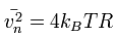where kB is Boltzmann's constant in joules per kelvin, T is the resistor's absolute temperature in kelvins, and R is the resistor value in ohms (Ω). Use this equation for quick calculation: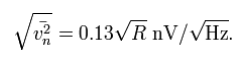For example, a 1 kΩ resistor at a temperature of 300 K has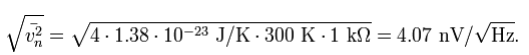For a given bandwidth, the root mean square (RMS) of the voltage, vn, is given by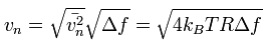where Δf is the bandwidth in hertz over which the noise is measured. For a 1 kΩ resistor at room temperature and a 10 kHz bandwidth, the RMS noise voltage is 400 nV. A useful rule of thumb to remember is that 50 Ω at 1 Hz bandwidth correspond to 1 nV noise at room temperature.

A resistor in a short circuit dissipates a noise power of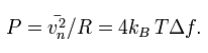The noise generated at the resistor can transfer to the remaining circuit; the maximum noise power transfer happens with impedance matching when the Thevenin equivalent resistance of the remaining circuit is equal to the noise generating resistance. In this case each one of the two participating resistors dissipates noise in both itself and in the other resistor. Since only half of the source voltage drops across any one of these resistors, the resulting noise power is given by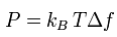where P is the thermal noise power in watts. Notice that this is independent of the noise generating resistance.

Noise current:

The noise source can also be modeled by a current source in parallel with the resistor by taking the Norton equivalent that corresponds simply to divide by R. This gives the root mean square value of the current source as: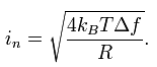Thermal noise is intrinsic to all resistors and is not a sign of poor design or manufacture, although resistors may also have excess noise.

Noise power in decibels:

Signal power is often measured in dBm (decibels relative to 1 milliwatt, assuming a 50 ohm load). From the equation above, noise power in a resistor at room temperature, in dBm, is then: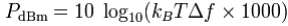where the factor of 1000 is present because the power is given in milliwatts, rather than watts. This equation can be simplified by separating the constant parts from the bandwidth: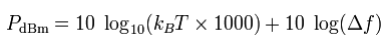which is more commonly seen approximated as: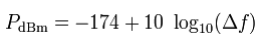Noise power at different bandwidths is then simple to calculate:

Bandwidth (Δf) Thermal noise power                              Notes

1 Hz −174 dBm

10 Hz −164 dBm

100 Hz −154 dBm

1 kHz −144 dBm

10 kHz −134 dBm                                                       FM channel of 2-way radio

100 kHz −124 dBm

180 kHz −121.45 dBm                                                 One LTE resource block

200 kHz −120.98 dBm                                                 One GSM channel (ARFCN)

1 MHz −114 dBm

2 MHz −111 dBm                                                             Commercial GPS channel

6 MHz −106 dBm                                                              Analog television channel

20 MHz −101 dBm                                                             WLAN 802.11 channel

Thermal noise on capacitors:

Thermal noise on capacitors is referred to as kTC noise. Thermal noise in an RC circuit has an unusually simple expression, as the value of the resistance (R) drops out of the equation. This is because higher R contributes to more filtering as well as to more noise. The noise bandwidth of the RC circuit is 1/(4RC), which can substituted into the above formula to eliminate R. The mean-square and RMS noise voltage generated in such a filter are: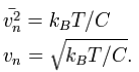Thermal noise accounts for 100% of kTC noise, whether it is attributed to the resistance or to the capacitance.

In the extreme case of the reset noise left on a capacitor by opening an ideal switch, the resistance is infinite, yet the formula still applies; however, now the RMS must be interpreted not as a time average, but as an average over many such reset events, since the voltage is constant when the bandwidth is zero. In this sense, the Johnson noise of an RC circuit can be seen to be inherent, an effect of the thermodynamic distribution of the number of electrons on the capacitor, even without the involvement of a resistor. The noise is not caused by the capacitor itself, but by the thermodynamic equilibrium of the amount of charge on the capacitor.

Once the capacitor is disconnected from a conducting circuit, the thermodynamic fluctuation is frozen at a random value with standard deviation as given above. The reset noise of capacitive sensors is often a limiting noise source, for example in image sensors. As an alternative to the voltage noise, the reset noise on the capacitor can also be quantified as the electrical charge standard deviation, as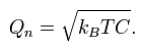Since the charge variance is kBTC, this noise is often called kTC noise.

Any system in thermal equilibrium has state variables with a mean energy of kT/2 per degree of freedom. Using the formula for energy on a capacitor (E = ½CV2 ), mean noise energy on a capacitor can be seen to also be ½C(kT/C), or also kT/2. Thermal noise on a capacitor can be derived from this relationship, without consideration of resistance.

The kTC noise is the dominant noise source at small capacitors.

Noise of capacitors at 300 K

Capacitance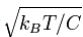Electrons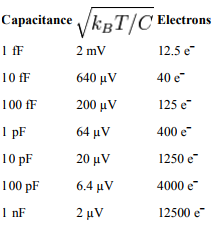Noise at very high frequencies:

The above equations are good approximations at any practical radio frequency in use (i.e. frequencies below about 80 gigahertz). In the most general case, which includes up to optical frequencies, the power spectral density of the voltage across the resistor R, in V2 /Hz is given by: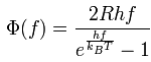where f is the frequency, h Planck's constant, kB Boltzmann constant and T the temperature in kelvins. If the frequency is low enough, that means: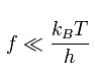(this assumption is valid until few terahertz at room temperature) then the exponential can be expressed in terms of its Taylor series. The relationship then becomes: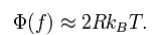In general, both R and T depend on frequency. In order to know the total noise it is enough to integrate over all the bandwidth. Since the signal is real, it is possible to integrate over only the positive frequencies, then multiply by 2. Assuming that R and T are constants over all the bandwidth Δf, then the root mean square (RMS) value of the voltage across a resistor due to thermal noise is given by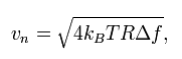that is, the same formula as above.

Offer running on EduRev: Apply code STAYHOME200 to get INR 200 off on our premium plan EduRev Infinity!

,

,

,

,

,

,

,

,

,

,

,

,

,

,

,

,

,

,

,

,

,

;